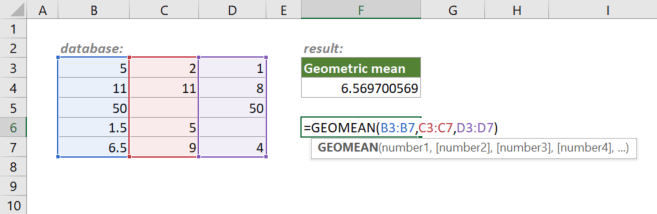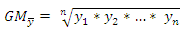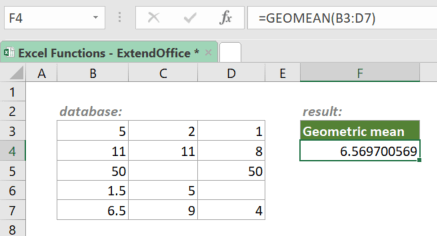## Excel GEOMEAN Function

The GEOMEAN function returns the geometric mean of an array or range of positive numbers. The geometric mean can be used for a set of numbers whose values are meant to be multiplied together or are exponential in nature, e.g., a set of growth figures.#### Syntax

=GEOMEAN(number1, [number2], ...)

#### Arguments

• number1 (required): The first positive number or range of positive numbers for which to calculate the geometric mean.
• [number2], ... (optional): The second and up to 253 more positive numbers or ranges of positive numbers for which to calculate the geometric mean.

#### Return Value

The GEOMEAN function returns a positive numeric value.

#### Function Notes

• Arguments can be numbers, arrays, or references that contain numbers.
• GEOMEAN ignores blank cells.
• If a text value is referenced by the GEOMEAN function, GEOMEAN will ignore it; If the text value is supplied directly to the GEOMEAN (e.g., =GEOMEAN(“kutools”)), the function will return the #VALUE! error.
• If a logical value is referenced by the GEOMEAN function, GEOMEAN will ignore it; If the logical value is supplied directly to the GEOMEAN, the function will treat is as a number (TRUE=1, FALSE=0).
• If any supplied values are not positive (≤ 0), GEOMEAN will return the #NUM! error value.
• If there are any error values in any of the arguments, GEOMEAN will return the first error value.
• The equation for the geometric mean is:#### Example

Supposing you have a table with numbers as shown below, to get the geometric mean of the numbers, please copy or enter one of the formulas below in a blank cell, and press Enter to get the result:

=GEOMEAN(5,2,1,11,11,8,50,50,1.5,5,6.5,9,4)

=GEOMEAN({5,2,1;11,11,8;50,50;1.5,5;6.5,9,4})

Or, use cell references to make the formulas dynamic:

=GEOMEAN(B3:D7)

=GEOMEAN(B3:B7,C3:C7,D3:D7)#### Related functions

Excel HARMEAN Function

The HARMEAN function returns the harmonic mean of a supplied set of values. The harmonic mean is the reciprocal of the arithmetic mean of reciprocals.

Excel TRIMMEAN Function

The Excel TRIMMEAN function calculates mean (average) while excluding outliers. The number of data points to exclude is provided as a percentage.

### The Best Office Productivity Tools

#### Kutools for Excel - Helps You To Stand Out From Crowd

 Popular Features: Find, Highlight or Identify Duplicates  |  Delete Blank Rows  |  Combine Columns or Cells without Losing Data  |  Round without Formula ... Super VLookup: Multiple Criteria  |  Multiple Value  |  Across Multi-Sheets  |  Fuzzy Lookup... Adv. Drop-down List: Easy Drop Down List  |  Dependent Drop Down List  |  Multi-select Drop Down List... Column Manager: Add a Specific Number of Columns  |  Move Columns  |  Toggle Visibility Status of Hidden Columns  |  Compare Columns to Select Same & Different Cells ... Featured Features: Grid Focus  |  Design View  |  Big Formula Bar  |  Workbook & Sheet Manager | Resource Library (Auto Text)  |  Date Picker  |  Combine Worksheets  |  Encrypt/Decrypt Cells  |  Send Emails by List  |  Super Filter  |  Special Filter (filter bold/italic/strikethrough...) ... Top 15 Toolsets:  12 Text Tools (Add Text, Remove Characters ...)  |  50+ Chart Types (Gantt Chart ...)  |  40+ Practical Formulas (Calculate age based on birthday ...)  |  19 Insertion Tools (Insert QR Code, Insert Picture from Path ...)  |  12 Conversion Tools (Numbers to Words, Currency Conversion ...)  |  7 Merge & Split Tools (Advanced Combine Rows, Split Excel Cells ...)  |  ... and more

Kutools for Excel Boasts Over 300 Features, Ensuring That What You Need is Just A Click Away...#### Office Tab - Enable Tabbed Reading and Editing in Microsoft Office (include Excel)

• One second to switch between dozens of open documents!
• Reduce hundreds of mouse clicks for you every day, say goodbye to mouse hand.
• Increases your productivity by 50% when viewing and editing multiple documents.
• Brings Efficient Tabs to Office (include Excel), Just Like Chrome, Edge and Firefox.